# RS Aggarwal Class 8 Solutions Chapter 10 Profit and Loss Ex 10D

## RS Aggarwal Class 8 Solutions Chapter 10 Profit and Loss Ex 10D

These Solutions are part of RS Aggarwal Solutions Class 8. Here we have given RS Aggarwal Solutions Class 8 Chapter 10 Profit and Loss Ex 10D.

Other Exercises

Question 1.
Solution:
C.P. of toy Rs. = 75
S.P. = Rs. 100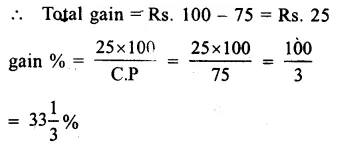Question 2.
Solution:
C.P. of bat = Rs. 120
S.P. = Rs. 105
Loss = Rs. 120 – Rs. 105 = Rs. 15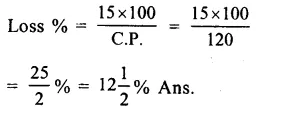Question 3.
Solution:
S.P. of book = Rs. 100
gain = Rs. 20
C.P. = 100 – 20 = Rs. 80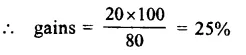Question 4.
Solution:
SP of an article = Rs. 48
Loss = 20%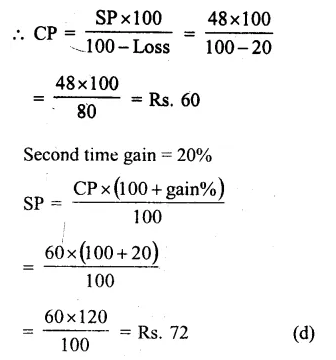Question 5.
Solution:
First time gain = 10%
Let SP – Rs. 100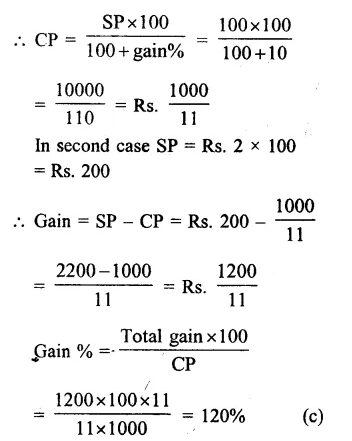Question 6.
Solution:
Let no. of bananas bought = 6
Now C.P. of bananas at the sale of 3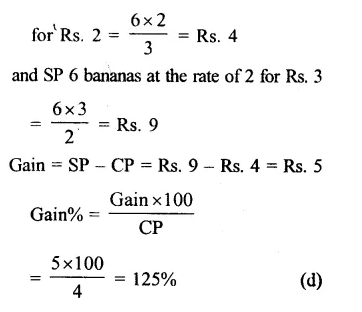Question 7.
Solution:
SP of 10 pens = CP of 12 pens
= Rs. 100 (suppose)Question 8.
Solution:
Gain on 100 pencils = SP of 20 pencils
SP of 100 pencils gains = CP of 100 pencils
=> SP of 100 pencils – SP of 20 pencils = CP of 100 pencils
=> SP of 80 pencils – CP of 100 pencils = Rs 100 (suppose)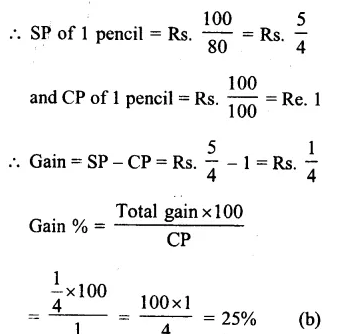Question 9.
Solution:
SP of 5 toffees = Re. 1
SP of 2 toffees = Re. 1
Now CP of 1 toffee = Rs. $$\\ \frac { 1 }{ 5 }$$
and SP of 1 toffee = Rs. $$\\ \frac { 1 }{ 2 }$$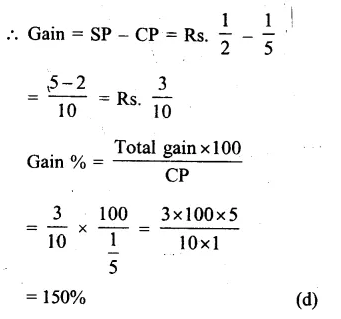Question 10.
Solution:
CP of 5 oranges = Rs. 10
SP of 6 oranges = Rs. 15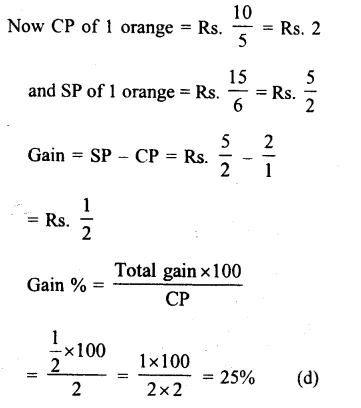Question 11.
Solution:
SP of a radio = Rs. 950
Loss = 5%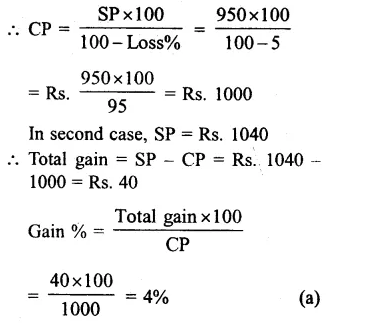Question 12.
Solution:
Let CP of an article = Rs. 100
SP = $$\\ \frac { 6 }{ 5 }$$ of CP = $$\\ \frac { 6 }{ 5 }$$ x 100 = Rs. 120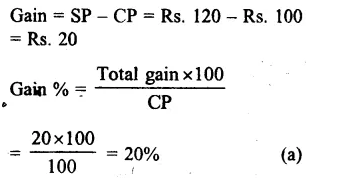Question 13.
Solution:
SP of a chair = Rs. 720
Loss = 25%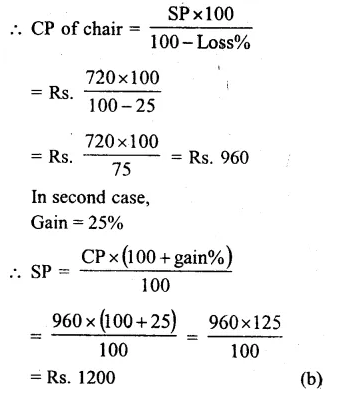Question 14.
Solution:
Ratio in CP and SP = 20 : 21
Let CP = Rs. 20
and SP = Rs. 21
Gain = SP – CP = Rs. 21 – 20 = Re. 1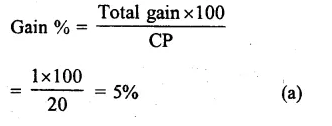Question 15.
Solution:
SP of first chair = Rs. 500
Gain = 20%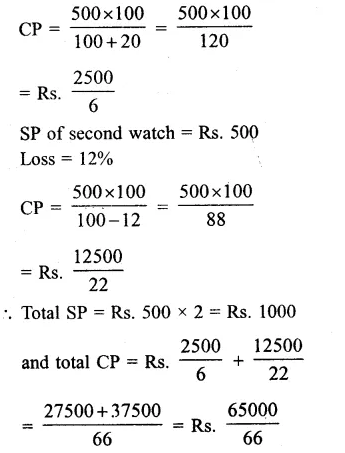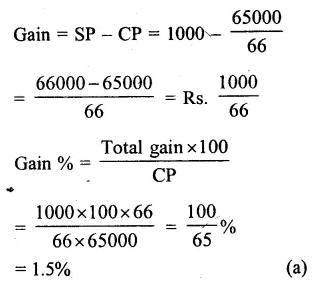Question 16.
Solution:
Gain % SP of Rs. 625 = Loss on SP of Rs. 435
CP of an article = x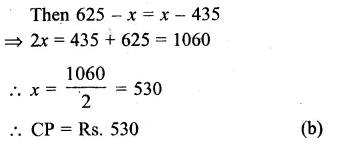Question 17.
Solution:
CP of an article = Rs. 150
Overhead expenses = 10% of CP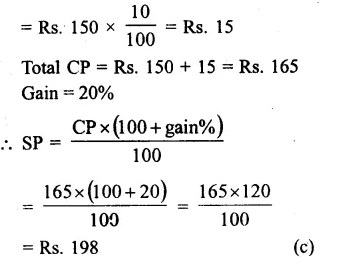Question 18.
Solution:
In first case, gain = 5%
and in second case, loss = 5%
and difference = Rs. 5 more
But difference in % = 5 + 5 = 10%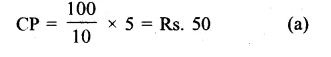Question 19.
Solution:
Let CP of an article = Rs. 100
List price = Rs. 100 + 20% of Rs. 100
= Rs. 100 + 20 = Rs. 120
Discount = 10%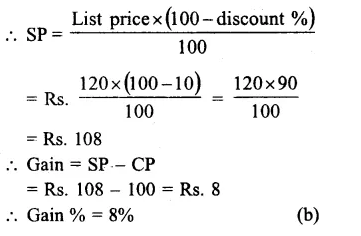Question 20.
Solution:
Let CP of an article = Rs. 100
Then Marked price
= Rs. 100 + 10% of 100
= Rs. 100 + 10 = Rs. 110
Discount = 10%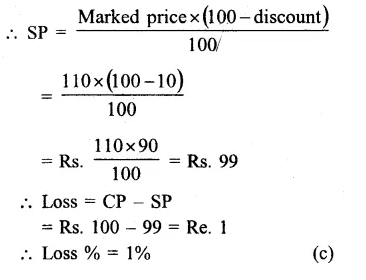Question 21.
Solution:
Price of watch including VAT = Rs. 825
VAT% = 10%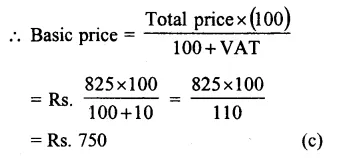Hope given RS Aggarwal Solutions Class 8 Chapter 10 Profit and Loss Ex 10D are helpful to complete your math homework.

If you have any doubts, please comment below. Learn Insta try to provide online math tutoring for you.